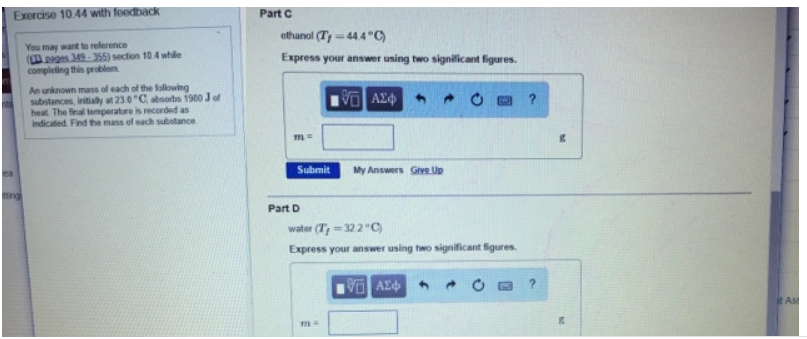# Problem: An unknown mass of each of the following substances, initially at 23°C, absorbs 1980 J of heat. The final temperature is recorded as indicated. Find the mass of each substance.Part Cethanol (Tf = 44.4°C) Express your answer using two significant figures. water (Tf = 32.2°C) Express your answer using two significant figures.

###### FREE Expert Solution###### Problem Details

An unknown mass of each of the following substances, initially at 23°C, absorbs 1980 J of heat. The final temperature is recorded as indicated. Find the mass of each substance.

Part C

ethanol (T= 44.4°C)